## Fit Fourier Models

A Fourier series describes a periodic function as a sum of sine and cosine functions. You can separate an arbitrary periodic function into simple components by using a Fourier series. These components are easy to integrate, differentiate, and analyze. For this reason, Fourier series are often used to approximate periodic signals.

Fourier series are represented in several forms. Curve Fitting Toolbox™ uses the trigonometric Fourier series form

`$y={a}_{0}+\sum _{i=1}^{n}{a}_{i}\mathrm{cos}\left(iwx\right)+{b}_{i}\mathrm{sin}\left(iwx\right)$`

where a0 models a constant (intercept) term in the data and is associated with the i = 0 cosine term, w is the fundamental frequency of the signal, and n is the number of terms (harmonics). Curve Fitting Toolbox supports Fourier series regression for 1 ≤ n ≤ 8.

### Fit Fourier Model Interactively in Curve Fitter App

This example shows how to use the Curve Fitter app to fit a Fourier model to data.

Load the sound signal sample data.

`load gong.mat`

The variables `y` and `Fs` contain sound signal and frequency data for a gong ring, respectively. Create a sound clip by storing the first 1000 elements of `y` in a vector named `gongClip`.

`gongClip = y(1:1000);`

To calculate the time corresponding to each element in `gongClip`, divide the index of the elements by `Fs`.

`t = [1:1000]./Fs;`

Open the Curve Fitter app from the command line.

`curveFitter`

Alternatively, on the Apps tab, in the Math, Statistics and Optimization group, click Curve Fitter.

In the Curve Fitter app, select the data variables for the fit. On the Curve Fitter tab, in the Data section, click Select Data. In the Select Fitting Data dialog box, select `gongClip` as the Y data value.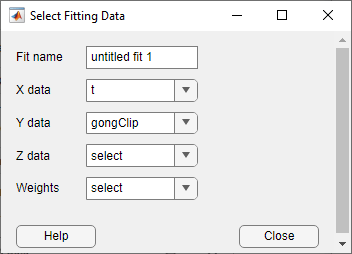The app plots the data points as you select variables. By default, the app fits a polynomial to the data. To fit a Fourier model, click `Fourier` in the Fit Type section of the Curve Fitter tab.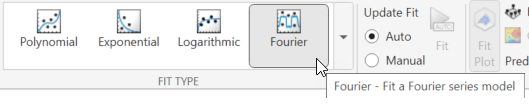The app fits a single-term Fourier model.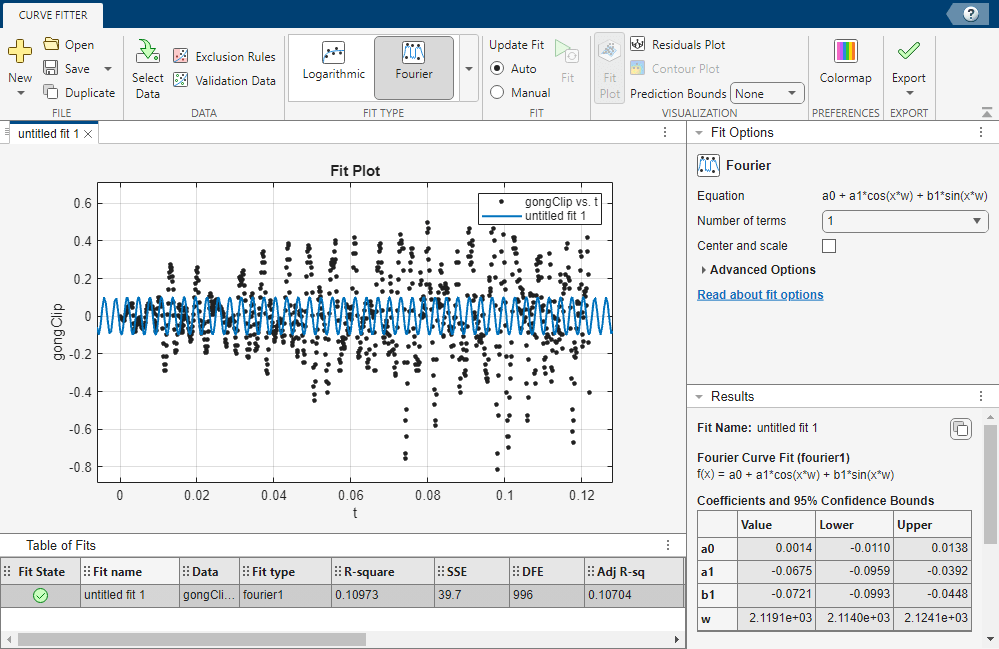The fitted one-term Fourier model is a periodic function with a simple oscillatory behavior. The Results panel displays the general equation for the model, fitted coefficient estimates with 95% confidence intervals, fundamental frequency, and goodness-of-fit statistics.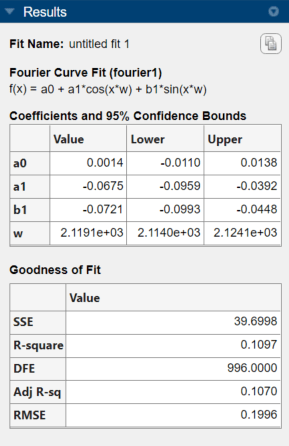The fitted one-term Fourier model has a root mean square error (RMSE) of 0.1996. To compare the one-term Fourier model with a Fourier model that has four terms, select `4` for Number of terms in the Fit Options panel. The app fits a Fourier model with four terms to the data.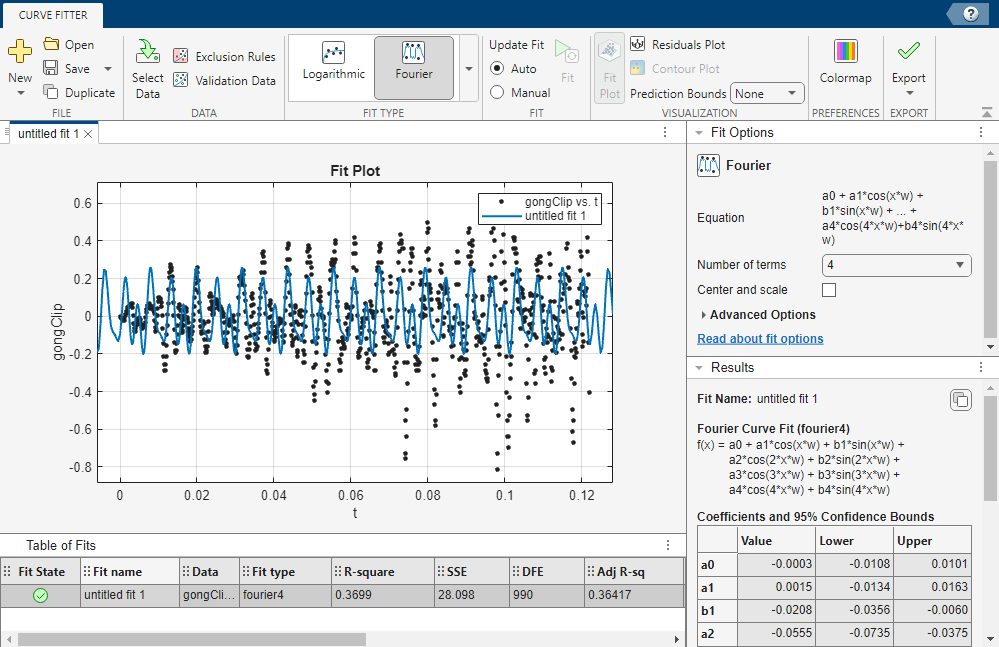The fitted four-term Fourier model has more complex oscillatory behavior than the one-term Fourier model. An RMSE of 0.1685 for the four-term model indicates that four terms predict the sound data more accurately than one. However, the plot shows that some of the data points in `gongClip` are outside of the range of the four-term model.

Export the fitted four-term Fourier model to the workspace by clicking Export in the Export section and then selecting `Export to Workspace`. In the dialog box, uncheck the second and third options. Store the fit in the variable name in the box next to the first option.You can listen to the sound data in `gongClip` by using the function `sound`.

```sound(gongClip,Fs) pause(2) % Allow gongClip to play before executing next line```

To get the sound data for the Fourier model approximation of `gongClip`, use `feval` to evaluate `gongFourierModel` at the times in `t`. Play the approximated sound data.

```gongClipApprox = feval(gongFourierModel,t); sound(gongClipApprox,Fs)```

The two clips have the same approximate average tone. However, the approximated sound data does not have as many fluctuations in tone as the sound data in `gongClip`.

### Fit Fourier Model at the Command Line

This example shows how to fit a Fourier model to data using the `fit` function.

Fit Two-Term Fourier Model

Load the El Niño-Southern Oscillation (ENSO) data.

`load enso;`

The variable `pressure` contains data for the averaged atmospheric pressure difference between Easter Island, Chile and Darwin, Australia. The variable `month` contains data for the month in which each pressure difference occurred.

Plot `pressure` against `month`.

`plot(month,pressure)`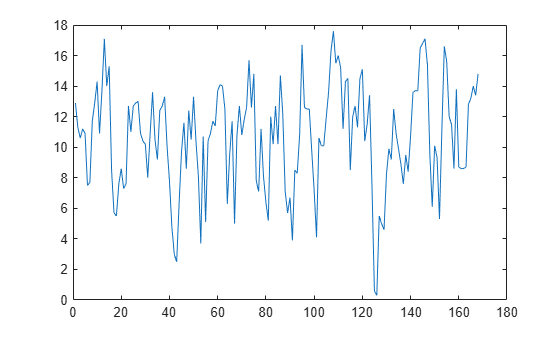The pressure data oscillates between 0 and 18, which indicates that it can be described by a Fourier series.

Fit a two-term Fourier model by using the Fourier library model. Specify the model type as `fourier` followed by the number of terms. Save the goodness-of-fit statistics for later comparison.

`[f2,gof2] = fit(month,pressure,"fourier2")`
```f2 = General model Fourier2: f2(x) = a0 + a1*cos(x*w) + b1*sin(x*w) + a2*cos(2*x*w) + b2*sin(2*x*w) Coefficients (with 95% confidence bounds): a0 = 10.63 (10.23, 11.03) a1 = 2.923 (2.27, 3.576) b1 = 1.059 (0.01593, 2.101) a2 = -0.5052 (-1.086, 0.07532) b2 = 0.2187 (-0.4202, 0.8576) w = 0.5258 (0.5222, 0.5294) ```
```gof2 = struct with fields: sse: 1.1230e+03 rsquare: 0.4279 dfe: 162 adjrsquare: 0.4103 rmse: 2.6329 ```

`f2` is a `cfit` object containing the general formula, coefficient estimates with 95% confidence bounds, and fundamental frequency for the fit `w`. The confidence bounds on `a2` and `b2` cross zero, so not enough evidence exists to conclude that they differ from zero or that the fitted model differs from a one-term Fourier model. The root mean square error (RMSE) of 2.6329 is useful for comparing the accuracy of `f2` to the accuracy of other fits.

To calculate the period from the fundamental frequency, use the formula `T = 2*pi/w`.

`w = f2.w`
```w = 0.5258 ```
`T = 2*pi/w`
```T = 11.9497 ```

The period of the fitted two-term Fourier model is approximately 12 months, or one year.

Plot `f2` with a scatter plot of the data.

`plot(f2,month,pressure)`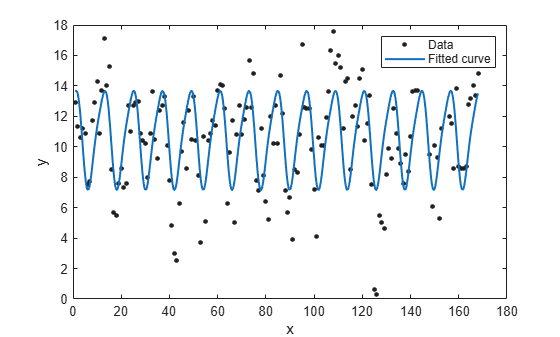The shape of `f2` is similar to the shape of a one-term Fourier model, and the oscillation peaks approximately once every 12 months.

Fit Seven-Term Fourier Model

Fit a seven-term Fourier model to the data. Save the goodness-of-fit statistics.

`[f7,gof7] = fit(month,pressure,"fourier7")`
```f7 = General model Fourier7: f7(x) = a0 + a1*cos(x*w) + b1*sin(x*w) + a2*cos(2*x*w) + b2*sin(2*x*w) + a3*cos(3*x*w) + b3*sin(3*x*w) + a4*cos(4*x*w) + b4*sin(4*x*w) + a5*cos(5*x*w) + b5*sin(5*x*w) + a6*cos(6*x*w) + b6*sin(6*x*w) + a7*cos(7*x*w) + b7*sin(7*x*w) Coefficients (with 95% confidence bounds): a0 = 10.63 (10.28, 10.97) a1 = 0.5669 (0.08285, 1.051) b1 = 0.1969 (-0.29, 0.6838) a2 = -1.203 (-1.687, -0.7189) b2 = -0.8085 (-1.307, -0.31) a3 = 0.9323 (0.4325, 1.432) b3 = 0.7599 (0.2622, 1.258) a4 = -0.6653 (-1.149, -0.1817) b4 = -0.2038 (-0.6995, 0.292) a5 = -0.02913 (-0.5129, 0.4547) b5 = -0.3701 (-0.8566, 0.1164) a6 = -0.04841 (-0.5437, 0.4469) b6 = -0.1367 (-0.6286, 0.3552) a7 = 2.812 (2.19, 3.433) b7 = 1.333 (0.4017, 2.264) w = 0.07527 (0.07478, 0.07576) ```
```gof7 = struct with fields: sse: 768.3656 rsquare: 0.6086 dfe: 152 adjrsquare: 0.5700 rmse: 2.2483 ```

`f7` contains several coefficients with confidence bounds that cross zero, so not enough evidence exists to conclude that their corresponding terms increase the accuracy of the fitted Fourier model. The RMSE of 2.2483 is smaller than the RMSE error of `f2`, which confirms that the seven-term Fourier model predicts the pressure more accurately than the two-term Fourier model.

To calculate the period from the fundamental frequency, use the formula T = `2*pi/w` to calculate the period.

`w = f7.w`
```w = 0.0753 ```
`T = (2*pi)/w`
```T = 83.4745 ```

The period of the fitted seven-term Fourier model is approximately 83 months, or roughly seven years. The amplitude of the fitted coefficients determines which terms contribute most to the predicted value of the pressure difference.

The period of a sinusoid of the form `sin(Ax)` or `cos(Ax)` is given by the formula T = `2*pi/|A|`. `a7` and `b7` are the largest coefficients.

`T = 2*pi/(w*7)`
```T = 11.9249 ```

The period of the terms corresponding to `a7` and `b7` is approximately 12 months, indicating that the annual cycle is the strongest.

Use the same formula to calculate the periods of the following terms:

• The terms `a1` and `b1` have a period of 7 years each.

• The terms `a2` and `b2` have a period of 3.5 (7/2) years each. The `a2` and `b2` coefficients have larger magnitude than a1 and b1, so the 3.5-year cycle contributes more to the predicted value of the pressure difference than the 7-year cycle.

• The terms `a3` and `b3` are strong, indicating 2.3-year (7/3) cycle.

Smaller terms such as `a6`, `b6`, `a5`, and `b5` are less important for the fit.

Plot `f7` with a scatter plot of the data.

`plot(f7,month,pressure)`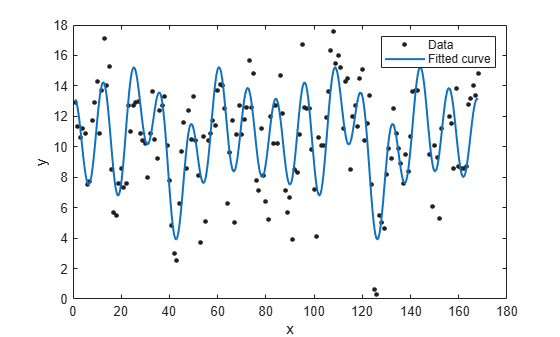The seven-term Fourier model oscillates in a more complex pattern and captures a wider range of values in the pressure difference than the one-term Fourier model. The cycle repeats approximately every 84 months, or 7 years. Typically, the El Niño warming happens at irregular intervals of two to seven years, and lasts nine months to two years. The average period length is five years. The model results reflect some of these periods.

Set Start Points

The `fit` function uses the `data` input argument to calculate optimized start points for the coefficient and fundamental frequency calculations. Fourier series models are particularly sensitive to start points, and the optimized values might be accurate for only a few terms in the associated equations. You can override the optimized start points by specifying the `StartPoint` name-value argument.

The extreme values in the scatter plot of the data suggest that a four-year cycle might be present. To confirm this suggestion, set the start point of the fundamental frequency to the value corresponding to a period of eight years, or 96 months. An eight-year period for the fitted Fourier model increases the period of the terms `a2` and `b2` from `3.5` to `4`.

`w_8 = (2*pi)/96`
```w_8 = 0.0654 ```

Find the index of the fundamental frequency in the cell vector of `f7` coefficient names by using the `coeffnames` function.

`coeffnames(f7)`
```ans = 16×1 cell {'a0'} {'a1'} {'b1'} {'a2'} {'b2'} {'a3'} {'b3'} {'a4'} {'b4'} {'a5'} {'b5'} {'a6'} {'b6'} {'a7'} {'b7'} {'w' } ```

The fundamental frequency is in the last entry of the vector of coefficient names. Create a vector of coefficient values from the coefficients of `f7`, and replace the value for the fundamental frequency with the value corresponding to an eight-year period.

```coeffs = coeffvalues(f7); coeffs(:,end) = w_8```
```coeffs = 1×16 10.6262 0.5669 0.1969 -1.2031 -0.8085 0.9323 0.7599 -0.6653 -0.2038 -0.0291 -0.3701 -0.0484 -0.1367 2.8120 1.3330 0.0654 ```

Fit a seven-term Fourier model to the pressure difference data using the coefficients with the new value for the fundamental frequency as the start point. Save the goodness-of-fit statistics.

`[f7_8,gof7_8] = fit(month,pressure,"fourier7",StartPoint=coeffs)`
```f7_8 = General model Fourier7: f7_8(x) = a0 + a1*cos(x*w) + b1*sin(x*w) + a2*cos(2*x*w) + b2*sin(2*x*w) + a3*cos(3*x*w) + b3*sin(3*x*w) + a4*cos(4*x*w) + b4*sin(4*x*w) + a5*cos(5*x*w) + b5*sin(5*x*w) + a6*cos(6*x*w) + b6*sin(6*x*w) + a7*cos(7*x*w) + b7*sin(7*x*w) Coefficients (with 95% confidence bounds): a0 = 10.58 (10.05, 11.1) a1 = 0.3286 (-0.4339, 1.091) b1 = -0.05917 (-0.7884, 0.6701) a2 = -0.8667 (-1.738, 0.004258) b2 = 1.094 (0.2819, 1.906) a3 = -0.4524 (-1.232, 0.3272) b3 = -0.3117 (-1.099, 0.4753) a4 = 0.181 (-0.7949, 1.157) b4 = 0.5806 (-0.1796, 1.341) a5 = 0.03263 (-0.7174, 0.7827) b5 = -0.2299 (-0.9767, 0.5169) a6 = 0.3726 (-0.39, 1.135) b6 = -0.2745 (-1.165, 0.6161) a7 = 0.4309 (-0.491, 1.353) b7 = -0.3547 (-1.316, 0.6062) w = 0.06795 (0.06519, 0.0707) ```
```gof7_8 = struct with fields: sse: 1.6851e+03 rsquare: 0.1416 dfe: 152 adjrsquare: 0.0568 rmse: 3.3296 ```

The coefficients of `f7_8` are slightly shifted from the `f7` coefficients. The higher RMSE for `f7_8` indicates that `f7` is a better fit for the data. Plot both fits to visually compare the models.

```plot(f7_8,month,pressure) hold on plot(f7, 'b') hold off legend("Data","f7_8","f7")```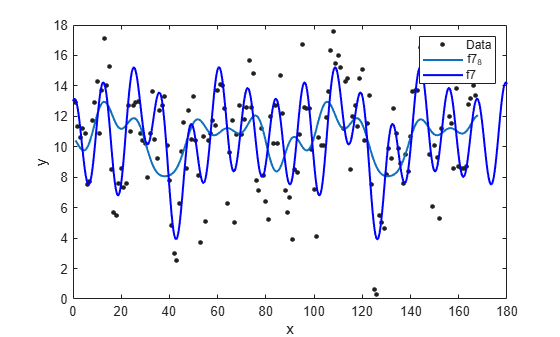The plot shows that `f7` captures the variation in the pressure difference data more accurately than `f7_8`.

Display Fourier Fit Iterations

An alternative to specifying additional options using name-value arguments is to pass a `fitoptions` object to the `fit` function. To view the available options for a Fourier model fit, pass the model name as an input argument to the `fitoptions` function.

`fitoptions("fourier7")`
```ans = Normalize: 'off' Exclude: [] Weights: [] Method: 'NonlinearLeastSquares' Robust: 'Off' StartPoint: [1×0 double] Lower: [1×0 double] Upper: [1×0 double] Algorithm: 'Trust-Region' DiffMinChange: 1.0000e-08 DiffMaxChange: 0.1000 Display: 'Notify' MaxFunEvals: 600 MaxIter: 400 TolFun: 1.0000e-06 TolX: 1.0000e-06 ```

Create a `fitoptions` object, and specify to display the output after each iteration.

`optionsf7 = fitoptions("fourier7",Display="iter")`
```options = Normalize: 'off' Exclude: [] Weights: [] Method: 'NonlinearLeastSquares' Robust: 'Off' StartPoint: [1×0 double] Lower: [1×0 double] Upper: [1×0 double] Algorithm: 'Trust-Region' DiffMinChange: 1.0000e-08 DiffMaxChange: 0.1000 Display: 'Iter' MaxFunEvals: 600 MaxIter: 400 TolFun: 1.0000e-06 TolX: 1.0000e-06 ```

`optionsf7` is a `fitoptions` object that contains the options for a seven-term Fourier model fit.

To view the iteration steps involved in creating `f7`, fit another seven-term Fourier model using the options in `optionsf7`.

`f7_iter = fit(month,pressure,"fourier7",optionsf7)`
``` Norm of First-order Iteration Func-count f(x) step optimality CG-iterations 0 2 768.41 1.93e+03 1 4 768.366 2.2176e-05 69.1 0 2 6 768.366 7.94962e-07 2.48 0 Success, but fitting stopped because change in residuals less than tolerance (TolFun). ```
```f7_iter = General model Fourier7: f7_iter(x) = a0 + a1*cos(x*w) + b1*sin(x*w) + a2*cos(2*x*w) + b2*sin(2*x*w) + a3*cos(3*x*w) + b3*sin(3*x*w) + a4*cos(4*x*w) + b4*sin(4*x*w) + a5*cos(5*x*w) + b5*sin(5*x*w) + a6*cos(6*x*w) + b6*sin(6*x*w) + a7*cos(7*x*w) + b7*sin(7*x*w) Coefficients (with 95% confidence bounds): a0 = 10.63 (10.28, 10.97) a1 = 0.5669 (0.08285, 1.051) b1 = 0.1969 (-0.29, 0.6838) a2 = -1.203 (-1.687, -0.7189) b2 = -0.8085 (-1.307, -0.31) a3 = 0.9323 (0.4325, 1.432) b3 = 0.7599 (0.2622, 1.258) a4 = -0.6653 (-1.149, -0.1817) b4 = -0.2038 (-0.6995, 0.292) a5 = -0.02913 (-0.5129, 0.4547) b5 = -0.3701 (-0.8566, 0.1164) a6 = -0.04841 (-0.5437, 0.4469) b6 = -0.1367 (-0.6286, 0.3552) a7 = 2.812 (2.19, 3.433) b7 = 1.333 (0.4017, 2.264) w = 0.07527 (0.07478, 0.07576) ```

To investigate the Fourier model fit further, you can experiment with specifying different options available for the `NonlinearLeastSquares` fitting algorithm. See `fitoptions` for more information.